# Dirichlet variational problemunder specified boundary conditions, where the functionis defined on the boundary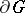of the-dimensional region. The solution of this problem is also the solution of the first boundary value problem for the Laplace equation: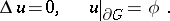It is natural to consider Dirichlet's variational problem in the class of functions with generalized first square-summable derivatives. If the region is bounded, this set of functions coincides with the Sobolev space, and is therefore complete in the appropriate metric. Moreover, each function in this space has boundary values onin the sense of almost-everywhere convergence which, if the boundary is sufficiently smooth, coincides with the boundary values in the sense of convergence in the mean or in the sense of the limit of the boundary values of functions continuous in the closed region which approximate the given function in the metric of the space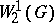. Ifis a bounded region and if there exists at least one functionfor which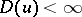and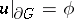(such functions are known as permissible), the solution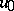of Dirichlet's variational problem exists and is unique. This solutionis a harmonic function in(cf. Dirichlet principle). If the boundaryofis smooth, then, for the class of permissible functions to be non-empty it is necessary and sufficient that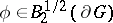. The solutionof Dirichlet's variational problem can be found by a direct variational method. These results are generalized to both the case of quadratic elliptic functionals containing higher-order derivatives and the case of unbounded regions.